# §5.2 3D编程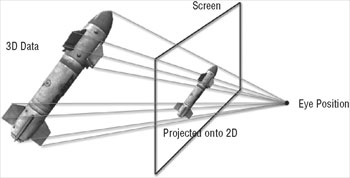BaseGame.WorldMatrix =Matrix.CreateRotationX(MathHelper.Pi / 2) *Matrix.CreateScale(2.5f) *Matrix.CreateTranslation(rocketPosition);

BaseGame.ViewMatrix =Matrix.CreateLookAt(cameraPosition, lookTarget, upVector);

/// <summary>

/// Field of view and near and far plane distances for the

/// ProjectionMatrix creation.

/// </summary>

private const float FieldOfView = (float)Math.PI / 2,NearPlane = 1.0f,FarPlane = 500.0f;…

aspectRatio = (float)width / (float)height;

BaseGame.ProjectionMatrix = Matrix.CreatePerspectiveFieldOfView(FieldOfView, aspectRatio, NearPlane, FarPlane);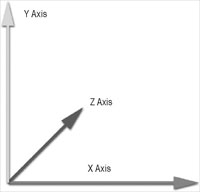你很可能从学校中记得左手坐标系以左手被命名，左手被用来展示给你X（拇指）、Y（食指）、Z（中指）轴......只要举起你的左手，这三个手指彼此校直90度，你就有了左手坐标系。在学校中，数学和物理都只使用右手坐标系，如果你用右手写字，举起你的左手来展示你这些轴，你会全部弄错，如果你在考试中的整个解决方案正好基于它就一点也不酷了。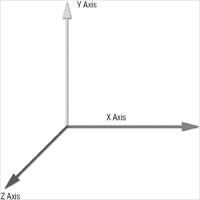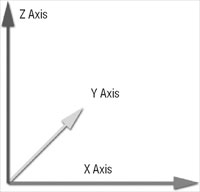public static void TestRenderModel() {

Model testModel = null;

TestGame.Start("TestRenderModel",delegate {

testModel = new Model("Rocket");

},

delegate{

testModel.Render(Matrix.CreateScale(10) );

}

);

} /} // TestRenderModel()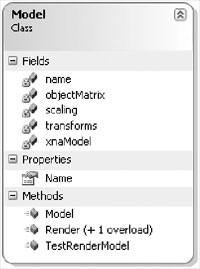/// <summary>

/// Create model

/// </summary>

/// <param name="setModelName" /> Set Model Filename

public Model(string setModelName) {

name = setModelName;

// Get matrices for each mesh part

transforms = new Matrix[xnaModel.Bones.Count];

xnaModel.CopyAbsoluteBoneTransformsTo(transforms);

// Calculate scaling for this object, used for rendering.

if (scaling == 0) scaling = 0.0001f;

// Apply scaling to objectMatrix to rescale object to size of 1.0

objectMatrix *= Matrix.CreateScale(1.0f / scaling);

} // Model(setModelName)

/// <param name="renderMatrix" /> Render matrix

public void Render(Matrix renderMatrix) {

// Apply objectMatrix

renderMatrix = objectMatrix * renderMatrix;

// Go through all meshes in the model

foreach (ModelMesh mesh in xnaModel.Meshes)

{

// Assign world matrix for each used effect

BaseGame.WorldMatrix =transforms[mesh.ParentBone.Index] *renderMatrix;

// And for each effect this mesh uses (usually just 1,multimaterials

// are nice in 3ds max, but not efficiently for rendering stuff).

foreach (Effect effect in mesh.Effects) {

// Set technique (not done automatically by XNA framework).

effect.CurrentTechnique = effect.Techniques["Specular20"];

// Set matrices, we use world, viewProj and viewInverse in

effect.Parameters["world"].SetValue(BaseGame.WorldMatrix);

// Note: these values should only be set once every frame!

// to improve performance again, also we should access them

// with EffectParameter and not via name (which is slower)!

effect.Parameters["viewProj"].SetValue(BaseGame.ViewProjectionMatrix);

effect.Parameters["viewInverse"].SetValue(BaseGame.InverseViewMatrix);

// Also set light direction (not really used here, but later)

effect.Parameters["lightDir"].SetValue(BaseGame.LightDirection);

}

// foreach (effect)

// Render with help of the XNA ModelMesh Draw method, which goes

// through all mesh parts and renders them (with vertex and index // buffers).

mesh.Draw();

} // foreach (mesh)

}

// Render(renderMatrix)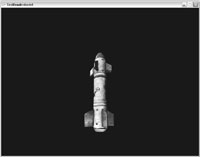## 测试其他模型Testing Other Models

public static void TestRenderModel() { "TestRenderModel",delegate {

testModel = new Model("Apple");

},

delegate {

testModel.Render(Matrix.CreateScale(10));

}

);

} // TestRenderModel()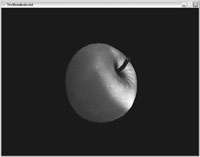2006 - 2023，推荐分辨率1024*768以上，推荐浏览器Chrome、Edge等现代浏览器，截止2021年12月5日的访问次数：1872万9823 站长邮箱